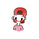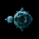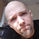2629 visualizzazioni
2629
Description:
• Designed for quick and clear visual analysis of market direction, aiming to high-light areas suitable for entry/exit
• Channel width's are based on the average high and low difference over X period, and as such can help determine reasonable target price and stop distances.
• There is no specific strategy to use with this indicator, it is only intended to help bolster confidence in your existing methods, especially for trend traders and scalpers.
Usage/setup:
• Option to use alternate time-frame.
• Multitude of MA types available for the basis, and relevant inputs.
• High-Low difference period - longer is smoother, but shorter will more accurately gauge current market variance.
• Colour swap delay period - Basis MA value must change up/down beyond average for X period in order for change to occur.
• % high-low channel values - a value of 1.0 is equal to 100% of the average high-low difference for selected basis period.
```//@version=2

study(title="High Low Difference Trend Assist", shorttitle="HLD Trend Assist", overlay=true)

// Revision:    1
// Author:      JayRogers
//
// Description:
//  - Designed for quick and clear visual analysis of market direction, aiming to high-light areas suitable for entry/exit
//  - Channel width's are based on the average high and low difference over X period, and as such can help determine
//    reasonable target price and stop distances.
//  - There is no specific strategy to use with this indicator, it is only intended to help bolster confidence in your
//    existing methods, especially for trend traders and scalpers.
// Usage/setup:
//  - Option to use alternate time-frame.
//  - Multitude of MA types available for the basis, and relevant inputs.
//  - High-Low difference period - longer is smoother, but shorter will more accurately guage current market variance.
//  - Colour swap delay period - Basis MA value must change up/down beyond average for X period in order for change to occur.
//  - % high-low channel values - a value of 1.0 is equal to 100% of the average high-low difference for selected basis period.

// === INPUTS ===
useRes      = input(defval = false, title = "Use Alternate Resolution?")
channelRes  = input(defval = "60", title = "Resolution", type = resolution)
basisType   = input(defval = "HullMA", title = "Basis Type: SMA, EMA, DEMA, TEMA, WMA, VWMA, SMMA, HullMA, LSMA, ALMA ( case sensitive )", type = string)
basisSource = input(defval = open, title = "Basis Source", type=source)
length1     = input(defval = 50, title = "Basis Period", minval = 1)
length2     = input(defval = 200, title = "High-Low Average Difference Period (higher is smoother)", minval = 1)
offSig      = input(defval = 6, title = "Offset for LSMA / Sigma for ALMA", minval = 0)
offsetALMA  = input(defval = 0.85, title = "Offset for ALMA", minval = 0, step = 0.01)
colSwitch   = input(defval = 7, title = "Basis MA Colour Swap Delay", minval = 0)
mult1       = input(defval = 2.0, title = "Center Channel Width (% high-low)", minval = 0.05, step = 0.05)
mult2       = input(defval = 3.0, title = "Outer Channels Offset (% high-low)", minval = 1, step = 0.05)
mult3       = input(defval = 1.5, title = "Outer Channels Width (% high-low)", minval = 0.1, step = 0.05)
// === /INPUTS ===

// === BASE FUNCTIONS ===
// Returns MA input selection variant, default to SMA if blank or typo.
variant(type, src, len, offSig, almaOffset) =>
v1 = sma(src, len)                                                  // Simple
v2 = ema(src, len)                                                  // Exponential
v3 = 2 * v2 - ema(v2, len)                                          // Double Exponential
v4 = 3 * (v2 - ema(v2, len)) + ema(ema(v2, len), len)               // Triple Exponential
v5 = wma(src, len)                                                  // Weighted
v6 = vwma(src, len)                                                 // Volume Weighted
v7 = na(v5) ? sma(src, len) : (v5 * (len - 1) + src) / len    // Smoothed
v8 = wma(2 * wma(src, len / 2) - wma(src, len), round(sqrt(len)))   // Hull
v9 = linreg(src, len, offSig)                                       // Least Squares
v10 = alma(src, len, almaOffset, offSig)                            // Arnaud Legoux
type=="EMA"?v2 : type=="DEMA"?v3 : type=="TEMA"?v4 : type=="WMA"?v5 : type=="VWMA"?v6 : type=="SMMA"?v7 : type=="HullMA"?v8 : type=="LSMA"?v9 : type=="ALMA"?v10 : v1
// security wrapper for repeat calls
reso(exp, use, res) => use ? security(tickerid, res, exp) : exp
// lagging average switch for MA direction
avgDir(exp, len) =>
average = len >= 2 ? sum(exp, len) / len : exp
up      = exp > average
down    = exp < average
state   = up ? true : down ? false : up
// === /BASE FUNCTIONS ===

// === SERIES VAR/FUNC ===
// setup base variables
midLine     = reso(variant(basisType, basisSource, length1, offSig, offsetALMA), useRes, channelRes)
midSwitch   = reso(avgDir(midLine, colSwitch), useRes, channelRes)
highLine    = reso(variant(basisType, high, length2, offSig, offsetALMA), useRes, channelRes)
lowLine     = reso(variant(basisType, low, length2, offSig, offsetALMA), useRes, channelRes)
highLowDiff = highLine - lowLine
// difference multiplier for band offsets
diffMult(mult) => midLine + (highLowDiff * mult)
// center channel bands
centerUpper = diffMult(mult1 / 2)
centerLower = diffMult(-(mult1 / 2))
// upper and lower bands
upperBand1  = diffMult(mult2)
upperBand2  = diffMult((mult2 + mult3))
lowerBand1  = diffMult(-mult2)
lowerBand2  = diffMult(-(mult2 + mult3))
// === /SERIES VAR/FUNC ===

// === STATE and ZONE LOGIC ===
// overall trend state and switch points
trendState  = open > centerUpper ? true : open < centerLower ? false : trendState
trendUp() => not trendState and trendState
trendDown() => trendState and not trendState
// upper channel zones
//zoneUpperMid(src) => src >= midLine and src <= centerUpper
//zoneUpperGap(src) => src >= centerUpper and src <= upperBand1
//zoneUpperOut(src) => src >= upperBand1
// lower channel zones
//zoneLowerMid(src) => src <= midLine and src >= centerLower
//zoneLowerGap(src) => src <= centerLower and src >= lowerBand1
//zoneLowerOut(src) => src <= lowerBand1
// === /STATE and ZONE LOGIC ===

// === PLOTTING ===
barcolor(color = trendState ? #009900 : #CC0000, title = "Trend Colours")
// basis line
p_mid   = plot(midLine, title = "Basis Line", color = midSwitch ? #009900 : #CC0000, linewidth = 3, style = line, transp = 20)
// central channel in up-trend
p_mid_u = plot(trendState ? midLine : na, transp = 100, editable = false)
p_cu_u  = plot(trendState ? centerUpper : na, title = "Central Channel Upper (in ut)", color = silver, transp = 100)
p_cl_u  = plot(trendState ? centerLower : na, title = "Central Channel Lower (in ut)", color = silver, transp = 100)
fill(p_cu_u, p_mid_u, title = "Central Channel Upper Fill (in ut)", color = silver, transp = 80)
fill(p_cl_u, p_mid_u, title = "Central Channel Lower Fill (in ut)", color = #009900, transp = 50)
// central channel in down-trend
p_mid_d = plot(trendState ? na : midLine, transp = 100, editable = false)
p_cu_d  = plot(trendState ? na : centerUpper, title = "Central Channel Upper (in dt)", color = silver, transp = 100)
p_cl_d  = plot(trendState ? na : centerLower, title = "Central Channel Lower (in dt)", color = silver, transp = 100)
fill(p_cu_d, p_mid_d, title = "Central Channel Upper Fill (in dt)", color = #CC0000, transp = 50)
fill(p_cl_d, p_mid_d, title = "Central Channel Lower Fill (in dt)", color = silver, transp = 80)
// upper offset channels
p_ub    = plot(upperBand1, title = "Outer Upper Band", color = #CC0000, linewidth = 2, style = line, transp = 40)
p_ubx   = plot(upperBand2, transp = 100, editable = false)
fill(p_ub, p_ubx, title = "Upper Channel Fill", color = silver, transp = 80)
// lower offset channels
p_lb    = plot(lowerBand1, title = "Outer Lower Band", color = #009900, linewidth = 2, style = line, transp = 40)
p_lbx   = plot(lowerBand2, transp = 100, editable = false)
fill(p_lb, p_lbx, title = "Lower Channel Fill", color = silver, transp = 80)
// === /PLOTTING ===```I would like to ask how to set the color change alarm, thank you, I am your fan
RispondiI like the fact you include LSMA in your work, highly overlooked tool. Thanks for sharing.
Rispondicoondawg71
Everyone has their own brand and flavour, and after I first wrote my MA multi select function i see little point for the majority of my projects in limiting selection. If I can squeeze a big extra chunk of potential functionality out of something just by dropping it in, why not eh? :) Saves any need for a re-write just to use a different MA type.
Rispondi